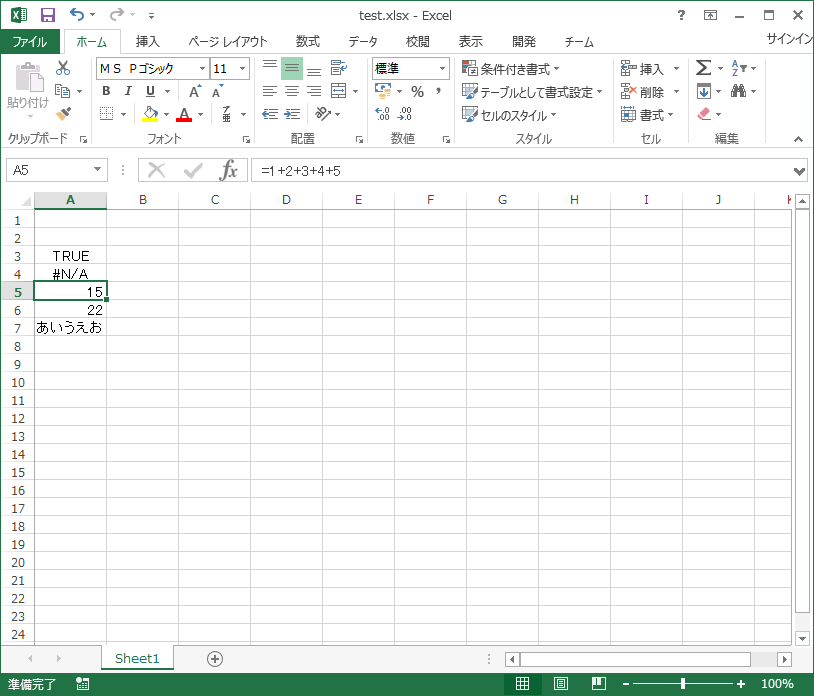# Importing Excel data in Java 2

Continuing from the last time, this time is also the contents of Apache POI.

• Last time https://qiita.com/Mk-4000/items/908266355307bcaf6993

About the part that was described in another method about the part to get the value of the previous cell. It doesn't seem like the cell values are simply brought in as is. Excerpt from the previous method as it is.

#### `SamplePOI.java`

``````
private static String getCellStringValue(Cell cell) {
String retStr;
CellType cellType = cell.getCellType();
switch (cellType) {
case STRING:
retStr = cell.getStringCellValue();
break;
case NUMERIC:
retStr = String.valueOf(cell.getNumericCellValue());
break;
case BOOLEAN:
retStr = String.valueOf(cell.getBooleanCellValue());
break;
case FORMULA:
retStr = String.valueOf(cell.getCellFormula());
break;
case ERROR:
retStr = String.valueOf(cell.getErrorCellValue());
break;
default:
retStr = "";
break;
}
return retStr;
}
}
``````

Here, the type of cell to be acquired (variable type? State? In Java) is first determined. It seems that the method used for acquisition changes depending on the type.

You can get the cell type with the getCellType method. Specifically, there are the following seven. ・ ** CellType._NONE ** Unknown cell. I'm not sure, but it's a cell that rarely appears.

・ ** CellType.BLANK ** Blank cell.

・ ** CellType.BOOLEAN ** Boolean. For cells that ask TRUE, FALSE, or authenticity (such as "= A1 = A2").

・ ** CellType.ERROR ** error. Perhaps a calculation error is a cell that has an error in the formula.

• Cells such as "# N / A" and "# DIV / 0!"

・ ** CellType.FORMULA ** Formula. A cell that is calculated like "= A1 + B1" and displayed as the calculated value on the display. Since the formula itself can be obtained by using the getCellFormula method, "= A1 + B1" can be obtained as it is by converting to String.

・ ** CellType.NUMERIC ** Numerical value. A cell that contains numbers or dates.

・ ** CellType.STRING ** String. A cell whose content is text. If you used numbers as strings on Excel, the numbers will come here as well. (Should)

### Verification

#### `SamplePOI2.java`

``````

public class SamplePOI2 {

/*
*This process
*/
public static void main(String[] args) {
//Enter the full path of the Excel file you want to import here
String ExcelPath = "";

//Objects for Excel
Workbook wb;
Sheet sh;
Row row;
Cell cell;

//List to hold the acquired data
List<String> columnA_List = new ArrayList<>();

try (InputStream is = new FileInputStream(ExcelPath)) {

//Import the target Excel file into Java
wb = WorkbookFactory.create(is);
//Specify the first sheet of the target file
sh = wb.getSheetAt(0);
//Get the maximum row in the sheet
int rowMaxA = sh.getLastRowNum();

//Turn the loop for the maximum row and get the cell of column A as a String type
for (int i = 0; i <= rowMaxA; i++) {
row = sh.getRow(i);
if (row == null) {
continue;
}
cell = row.getCell(0);
String cellValue = getCellTypes(cell);
}

//Output to console

for (String outStr : columnA_List) {
System.out.println(outStr);
}

} catch (Exception e) {
e.printStackTrace();
}
}

/*
*Determines the state of the cell and returns it as a String type.
*/
private static String checkCellType(Cell cell) {
String retStr="";
CellType cellType = cell.getCellType();
switch (cellType) {
case _NONE:
retStr ="_NONE";
break;
case BLANK:
retStr ="BLANK";
break;
case BOOLEAN:
retStr ="BOOLEAN";
break;
case ERROR:
retStr ="ERROR";
break;
case FORMULA:
retStr ="FORMULA";
break;
case NUMERIC:
retStr ="NUMERIC";
break;
case STRING:
retStr ="STRING";
break;
}
return retStr;
}
``````The result of loading this Excel is

#### `cellType.result`

``````
BLANK
BOOLEAN
ERROR
FORMULA
NUMERIC
STRING
``````

have become.